# Electrochemistry ch 14

26 de Jun de 2014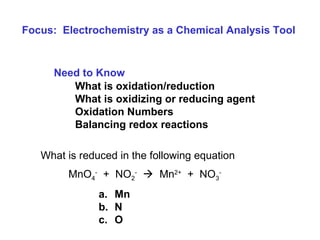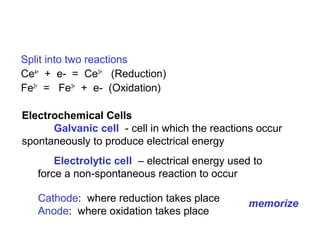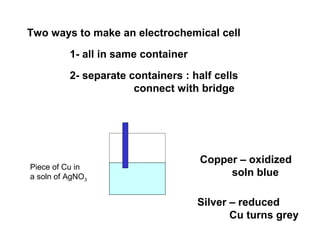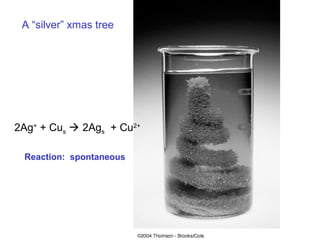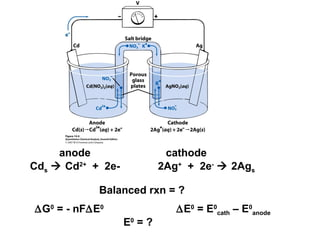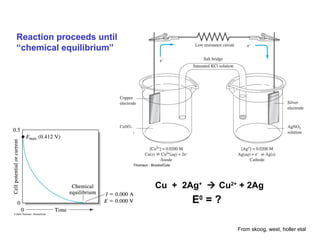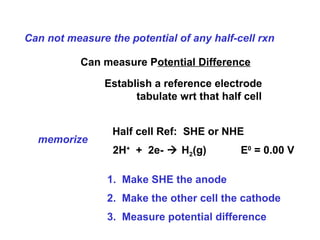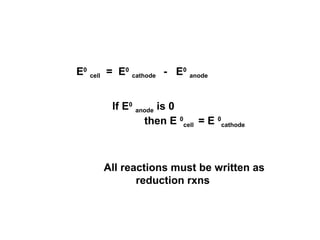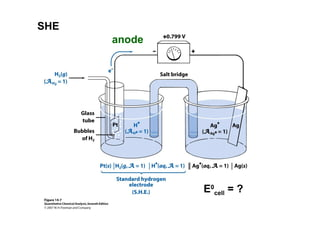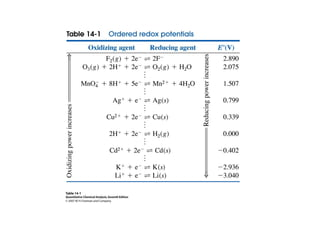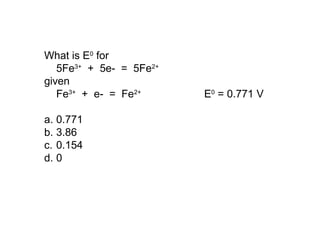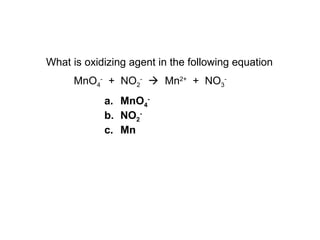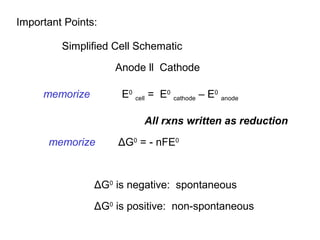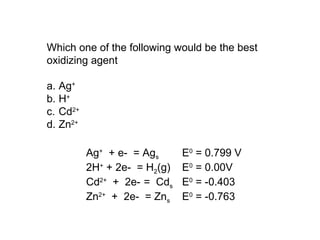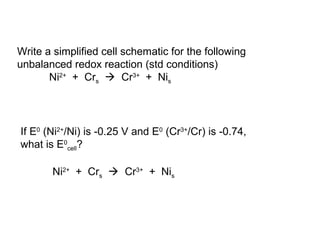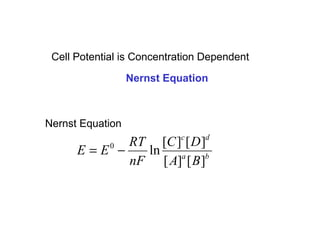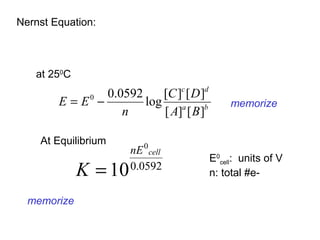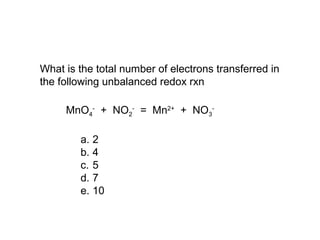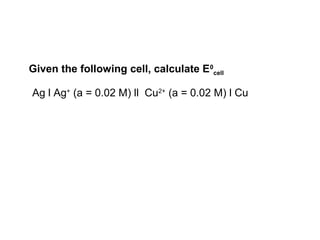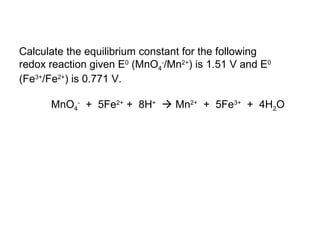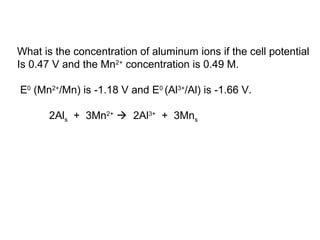1 de 22

### Electrochemistry ch 14

1. Need to Know What is oxidation/reduction What is oxidizing or reducing agent Oxidation Numbers Balancing redox reactions Focus: Electrochemistry as a Chemical Analysis Tool MnO4 - + NO2 -  Mn2+ + NO3 - What is reduced in the following equation a. Mn b. N c. O
2. Split into two reactions Ce4+ + e- = Ce3+ (Reduction) Fe2+ = Fe3+ + e- (Oxidation) Electrochemical Cells Galvanic cell - cell in which the reactions occur spontaneously to produce electrical energy Electrolytic cell – electrical energy used to force a non-spontaneous reaction to occur Cathode: where reduction takes place Anode: where oxidation takes place memorize
3. Two ways to make an electrochemical cell 1- all in same container 2- separate containers : half cells connect with bridge Copper – oxidized soln blue Silver – reduced Cu turns grey Piece of Cu in a soln of AgNO3
4. 2Ag+ + Cus  2Ags + Cu2+ A “silver” xmas tree Reaction: spontaneous
5. 2Ag+ + 2e-  2AgsCds  Cd2+ + 2e- anode cathode Balanced rxn = ? ∆G0 = - nF∆E0 ∆E0 = E0 cath – E0 anode E0 = ?
6. Reaction proceeds until “chemical equilibrium” Cu + 2Ag+  Cu2+ + 2Ag E0 = ? From skoog, west, holler etal
7. Can not measure the potential of any half-cell rxn Can measure Potential Difference Establish a reference electrode tabulate wrt that half cell Half cell Ref: SHE or NHE 1. Make SHE the anode 2. Make the other cell the cathode 3. Measure potential difference 2H+ + 2e-  H2(g) E0 = 0.00 V memorize
8. E0 cell = E0 cathode - E0 anode If E0 anode is 0 then E 0 cell = E 0 cathode All reactions must be written as reduction rxns
9. SHE anode E0 cell = ?
10. What is E0 for 5Fe3+ + 5e- = 5Fe2+ given Fe3+ + e- = Fe2+ E0 = 0.771 V a. 0.771 b. 3.86 c. 0.154 d. 0
11. MnO4 - + NO2 -  Mn2+ + NO3 - What is oxidizing agent in the following equation a. MnO4 - b. NO2 - c. Mn
12. Important Points: Simplified Cell Schematic Anode ll Cathode E0 cell = E0 cathode – E0 anode All rxns written as reduction ΔG0 = - nFE0 memorize memorize ΔG0 is negative: spontaneous ΔG0 is positive: non-spontaneous
13. Which one of the following would be the best oxidizing agent a. Ag+ b. H+ c. Cd2+ d. Zn2+ Ag+ + e- = Ags E0 = 0.799 V 2H+ + 2e- = H2(g) E0 = 0.00V Cd2+ + 2e- = Cds E0 = -0.403 Zn2+ + 2e- = Zns E0 = -0.763
14. Write a simplified cell schematic for the following unbalanced redox reaction (std conditions) Ni2+ + Crs  Cr3+ + Nis If E0 (Ni2+ /Ni) is -0.25 V and E0 (Cr3+ /Cr) is -0.74, what is E0 cell? Ni2+ + Crs  Cr3+ + Nis
15. Cell Potential is Concentration Dependent Nernst Equation ba dc BA DC nF RT EE ][][ ][][ ln0 −= Nernst Equation
16. Nernst Equation: at 250 C ba dc BA DC n EE ][][ ][][ log 0592.00 −= At Equilibrium 0592.0 0 10 cellnE K = E0 cell: units of V n: total #e- memorize memorize
17. What is the total number of electrons transferred in the following unbalanced redox rxn MnO4 - + NO2 - = Mn2+ + NO3 - a. 2 b. 4 c. 5 d. 7 e. 10
18. Given the following cell, calculate E0 cell Ag l Ag+ (a = 0.02 M) ll Cu2+ (a = 0.02 M) l Cu
19. Calculate the equilibrium constant for the following redox reaction given E0 (MnO4 - /Mn2+ ) is 1.51 V and E0 (Fe3+ /Fe2+ ) is 0.771 V. MnO4 - + 5Fe2+ + 8H+  Mn2+ + 5Fe3+ + 4H2O
20. What is the concentration of aluminum ions if the cell potential Is 0.47 V and the Mn2+ concentration is 0.49 M. E0 (Mn2+ /Mn) is -1.18 V and E0 (Al3+ /Al) is -1.66 V. 2Als + 3Mn2+  2Al3+ + 3Mns